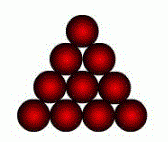Competitions

# Bob and balls

Time limit 1 second
Memory limit 128 MiB

Recently Bob learned that balls can be played in a very entertaining game. In this game you want to stack the balls in the form of various geometric shapes. Just now Bob is engaged in laying the balls in the form of an equilateral triangle. But here's the thing: sometimes Bob do not have enough balls, and he wants to know, what is the greatest side of the triangle for which it is enough balls? Help Bob to count the value of n - the length of equilateral triangle for given number of balls k.

Below given the example of placing the balls in the form of an equilateral triangle:## Input data

One positive integer k (0k2 *10^8) - the existing number of balls.

## Output data

Print the value of n - the answer to the problem.

## Examples

Input example #1
6

Output example #1
3

Input example #2
5

Output example #2
2

Author Евгений Симоненко
Source Дистанционная Летняя Компьютерная Школа - лето 2013 года